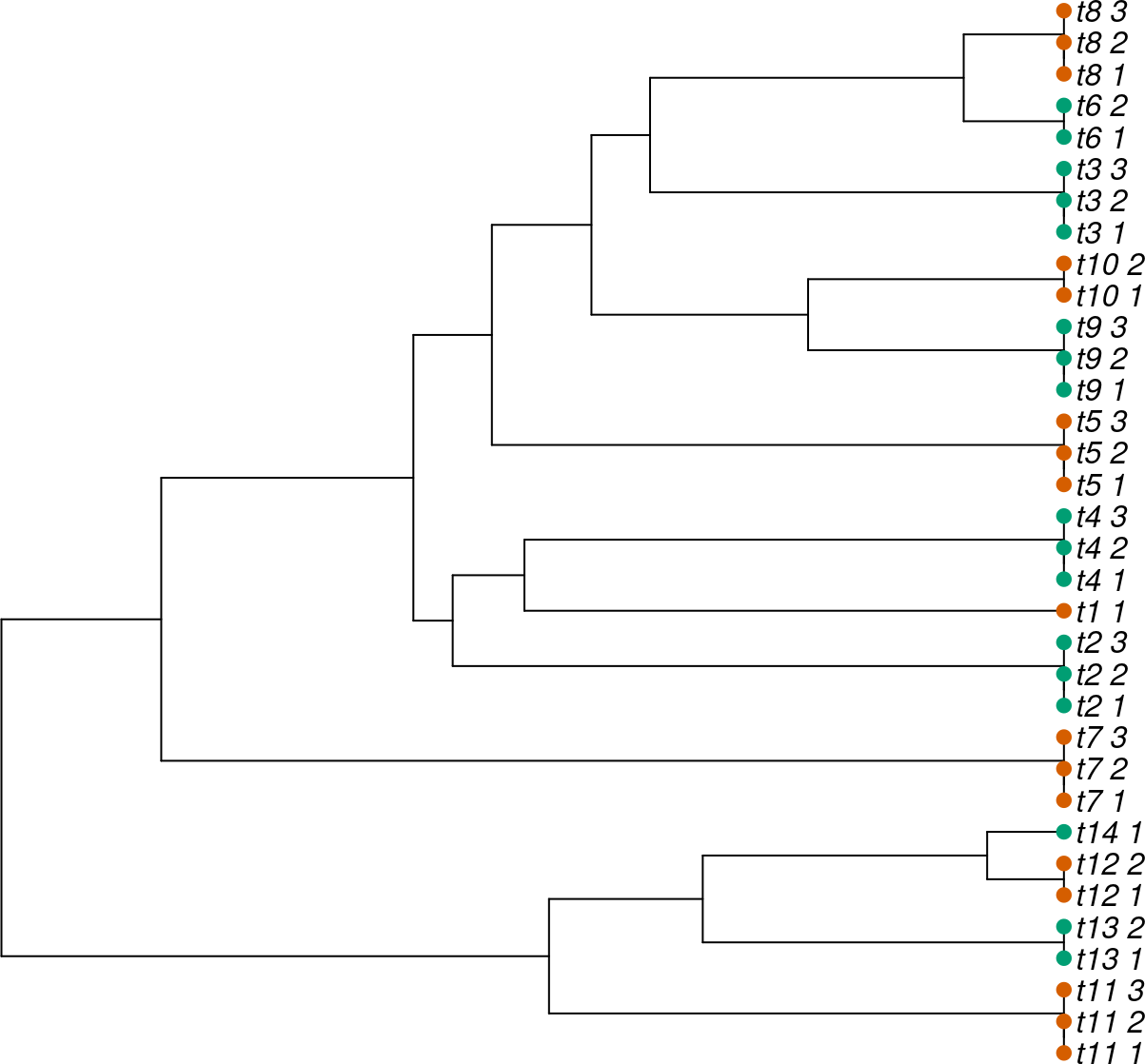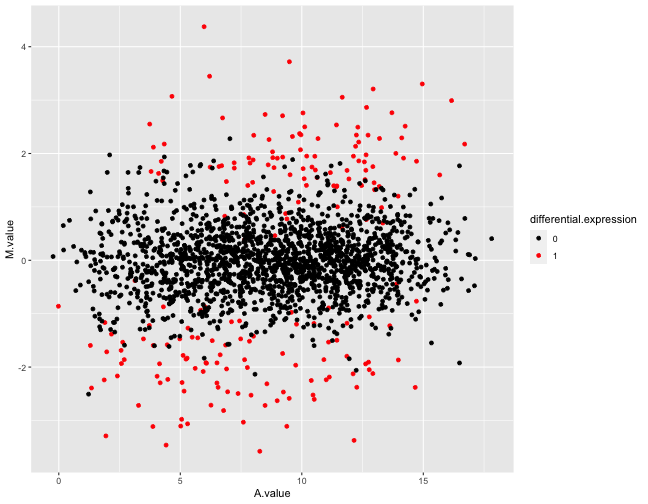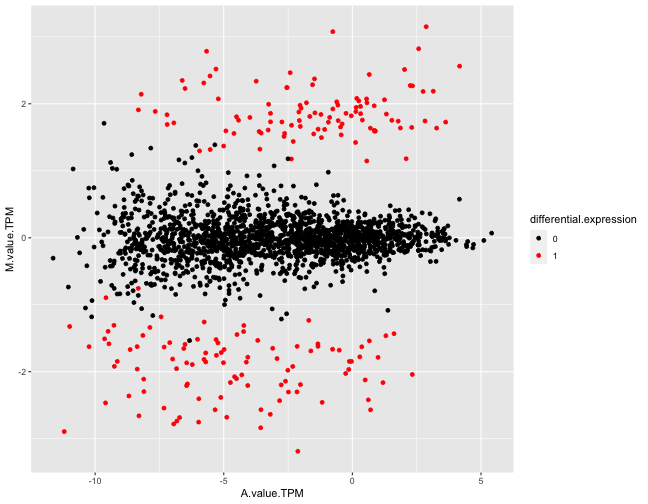# Contents

``library(compcodeR)``

# 1 Introduction

The compcodeR R package can generate RNAseq counts data and compare the relative performances of various popular differential analysis detection tools (Soneson and Delorenzi (2013)).

Using the same framework, this document shows how to generate “orthologous gene” (OG) expression for different species, taking into account their varying lengths, and their phylogenetic relationships, as encoded by an evolutionary tree.

This vignette provides a tutorial on how to use the “phylogenetic” functionalities of compcodeR. It assumes that the reader is already familiar with the `compcodeR` package vignette.

# 2 The `phyloCompData` class

The `phyloCompData` class extends the `compData` class of the compcodeR package to account for phylogeny and length information needed in the representation of OG expression data.

A `phyloCompData` object contains all the slots of a `compData` object, with an added slot containing a phylogenetic tree with `ape` format `phylo`, and a length matrix. It can also contain some added variable information, such as species names. More detailed information about the `phyloCompData` class are available in the section on the phylo data object. After conducting a differential expression analysis, the `phyloCompData` object has the same added information than the `compData` object (see the result object in the compcodeR package vignette).

# 3 A sample workflow

The workflow for working with the inter-species extension is very similar to the already existing workflow of the compcodeR package. In this section, we recall this workflow, stressing out the added functionalities.

## 3.1 Phylogenetic Tree

The simulations are performed following the description by Bastide et al. (2022).

We use here the phylogenetic tree issued from Stern et al. (2017), normalized to unit height, that has \(14\) species with up to 3 replicates, for a total number of sample equal to \(34\) (see Figure below).

``````library(ape)
tree <- system.file("extdata", "Stern2018.tree", package = "compcodeR")

Note that any other tree could be used, for instance randomly generated using a birth-death process, see e.g. function `rphylo` in the `ape` package.

## 3.2 Condition Design

To conduct a differential analysis, each species must be attributed a condition. Because of the phylogenetic structure, the condition design does matter, and have a strong influence on the data produced. Here, we assume that the conditions are mapped on the tree in a balanced way (“alt” design), which is the “best case scenario”.

``````# link each sample to a species
id_species <- factor(sub("_.*", "", tree\$tip.label))
names(id_species) <- tree\$tip.label
# Assign a condition to each species
species_names <- unique(id_species)
species_names[c(length(species_names)-1, length(species_names))] <- species_names[c(length(species_names), length(species_names)-1)]
cond_species <- rep(c(1, 2), length(species_names) / 2)
names(cond_species) <- species_names
# map them on the tree
id_cond <- id_species
id_cond <- cond_species[as.vector(id_cond)]
id_cond <- as.factor(id_cond)
names(id_cond) <- tree\$tip.label``````

We can plot the assigned conditions on the tree to visualize them.

``````plot(tree, label.offset = 0.01)
tiplabels(pch = 19, col = c("#D55E00", "#009E73")[id_cond])``````Figure 1: Phylogenetic tree with \(14\) species and \(34\) samples, with two conditions

## 3.3 Simulating data

Using this tree with associated condition design, we can then generate a dataset using a “phylogenetic Poisson Log Normal” (pPLN) distribution. We use here a Brownian Motion (BM) model of evolution for the latent phylogenetic log normal continuous trait, and assume that the phylogenetic model accounts for \(90\%\) of the latent trait variance (i.e. there is an added uniform intra-species variance representing \(10\%\) of the total latent trait variation). Using the `"auto"` setup, the counts are simulated so that they match empirical moments found in Stern and Crandall (2018). OG lengths are also drawn from a pPLN model, so that their moments match those of the empirical dataset of Stern and Crandall (2018). We choose to simulate \(2000\) OGs, \(10\%\) of which are differentially expressed, with an effect size of \(3\).

The following code creates a `phyloCompData` object containing the simulated data set and saves it to a file named `"alt_BM_repl1.rds"`.

``````set.seed(12890926)
alt_BM <- generateSyntheticData(dataset = "alt_BM",
n.vars = 2000, samples.per.cond = 17,
n.diffexp = 200, repl.id = 1,
seqdepth = 1e7, effect.size = 3,
fraction.upregulated = 0.5,
output.file = "alt_BM_repl1.rds",
## Phylogenetic parameters
tree = tree,                      ## Phylogenetic tree
id.species = id_species,          ## Species structure of samples
id.condition = id_cond,           ## Condition design
model.process = "BM",             ## The latent trait follows a BM
prop.var.tree = 0.9,              ## Tree accounts for 90% of the variance
lengths.relmeans = "auto",        ## OG length mean and dispersion
lengths.dispersions = "auto")     ## are taken from an empirical exemple``````

The `summarizeSyntheticDataSet` works the same way as in the base `compcodeR` package, generating a report that summarize all the parameters used in the simulation, and showing some diagnostic plots.

``````summarizeSyntheticDataSet(data.set = "alt_BM_repl1.rds",
output.filename = "alt_BM_repl1_datacheck.html")``````

When applied to a `phyloCompData` object, it provides some extra diagnostics, related to the phylogenetic nature of the data. In particular, it contains MA-plots with TPM-normalized expression levels to take OG length into account, which generally makes the original signal clearer.Figure 2: Example figures from the summarization report generated for a simulated data set
The top panel shows an MA plot, with the genes colored by the true differential expression status. The bottom panel shows the same plot, but using TPM-normalized estimated expression levels.

It also shows a log2 normalized counts heatmap plotted along the phylogeny, illustrating the phylogenetic structure of the differentially expressed OGs.